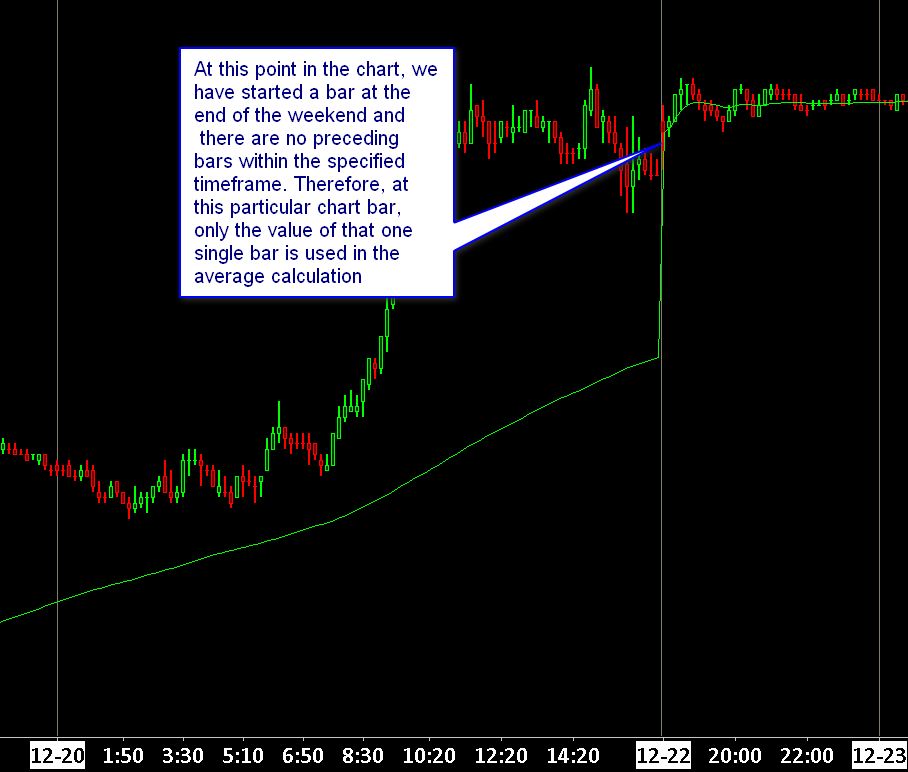# Technical Studies Reference

### Moving Average - Time Period

The Moving Average - Time Period study calculates a moving average using a Length specified by a fixed amount of time instead of a number of bars.

At each chart bar, the nearest matching bar with a Date-Time which is prior to the current bar Date-Time by the time period specified by the Time Period Type and Time Period Length Inputs, is where the calculation begins at.

The value of each element of a chart bars specified by the Input Data Input is summed except for zero values and divided by the number of nonzero values.

As you change the chart bar timeframe, you are going to get different results because the Input Data, like the last trade price of the bar, is going to be different for each chart bar. And the number of bars used in the calculation also varies based upon the timeframe of the chart bars.

If at a particular chart bar, based upon weekends and holidays and non-trading times, there are fewer bars than usual when looking back by the specified amount of time, then there are going to be fewer bar values used in the average calculation. Therefore, this can cause a sudden shift in the average value at that chart bar.

It is necessary that the chart loads at least the number of days that the Time Period Type and Time Period Length Input Settings specify. Refer to Days to Load in Chart Settings. Otherwise, this study cannot reference the appropriate amount of data required.

It is not possible to compare the result of Moving Average - Time Period to the result of Moving Average-Simple. For example, on a Historical Daily Chart when using Moving Average - Time Period and it has a Length Input of 100, this is using 100 days but the actual time span of days the study is actually using due to weekends and nontrading days is actually significantly longer. Therefore, if you set Moving Average - Time Period to reference 100 days, it is going to be a different result at each chart bar.